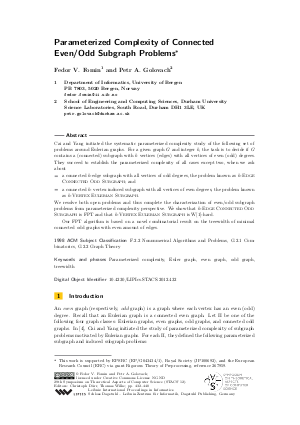Document# Parameterized Complexity of Connected Even/Odd Subgraph Problems

### Authors Fedor V. Fomin, Petr A. Golovach## File

LIPIcs.STACS.2012.432.pdf
• Filesize: 0.64 MB
• 9 pages

## Cite As

Fedor V. Fomin and Petr A. Golovach. Parameterized Complexity of Connected Even/Odd Subgraph Problems. In 29th International Symposium on Theoretical Aspects of Computer Science (STACS 2012). Leibniz International Proceedings in Informatics (LIPIcs), Volume 14, pp. 432-440, Schloss Dagstuhl - Leibniz-Zentrum für Informatik (2012)
https://doi.org/10.4230/LIPIcs.STACS.2012.432

## Abstract

Cai and Yang initiated the systematic parameterized complexity study of the following set of problems around Eulerian graphs. For a given graph G and integer k, the task is to decide if G contains a (connected) subgraph with k vertices (edges) with all vertices of even (odd) degrees. They succeed to establish the parameterized complexity of all cases except two, when we ask about - a connected k-edge subgraph with all vertices of odd degrees, the problem known as k-Edge Connected Odd Subgraph; and - a connected k- vertex induced subgraph with all vertices of even degrees, the problem known as k-Vertex Eulerian Subgraph. We resolve both open problems and thus complete the characterization of even/odd subgraph problems from parameterized complexity perspective. We show that k-Edge Connected Odd Subgraph is FPT and that k-Vertex Eulerian Subgraph is W-hard. Our FPT algorithm is based on a novel combinatorial result on the treewidth of minimal connected odd graphs with even amount of edges.
##### Keywords
• Parameterized complexity
• Euler graph
• even graph
• odd graph
• treewidth

## Metrics

• Access Statistics
• Total Accesses (updated on a weekly basis)
0